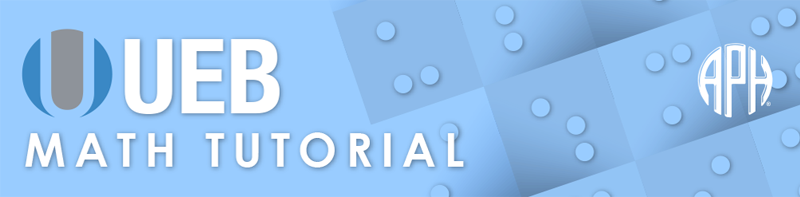Practice Problems -   -  Use 6 Dot Entry   Switch to Nemeth Tutorial

# Lesson 3.4: Omissions

## Symbols

$_\phantom{\rule{.3em}{0ex}}\text{low line underscore}$
⠨⠤

$\dots \phantom{\rule{.3em}{0ex}}\text{ellipsis}$
⠲⠲⠲

## Explanation

Math exercises and worksheets often present problems where the student is asked to fill in an omitted item or missing information. Omissions in print are represented by a blank space, low horizontal line, a question mark, an ellipsis, or a hollow shape. (Shapes will be covered in a later section.)

The symbol shown in print to represent an omission should be used in UEB. If the omission represents a missing number, then it is spaced as a number in the expression. If it is being used to represent an omitted sign of operation, then the spacing used for signs of operation applies. If it is used to indicate that a sign of comparison such as the equals sign is omitted, then there should be a space before and after the symbol used for the omission.

The visible space symbol is used when an omission is represented by a blank space in print. The visible space symbol is dots three four six. The symbol is spaced based on the item it represents.

In Examples 1 and 2 the omission is represented by a blank space. The omission in Example 1 is the sum for the problem 2 plus 4. In Example 2 a number is missing from the equation, shown as three plus space equals five. The visible space symbol is used and spaced accordingly.

⠼⠃⠐⠖⠼⠙⠀⠐⠶⠀⠬

### Example 2

⠼⠉⠐⠖⠬⠀⠐⠶⠀⠼⠑

A question mark is used to represent the omission in Example 3, which appears after the equals sign. Since the question mark stands alone in this example and its braille symbol also has a grade 2 meaning, the grade 1 indicator must be used.

### Example 3

$9+4=?$
⠼⠊⠐⠖⠼⠙⠀⠐⠶⠀⠰⠦

In Example 4 the question mark is used to represent an omitted numeral in an equation. The question mark does not stand alone therefore the grade 1 indicator is not needed.

### Example 4

$6×?=24$
⠼⠋⠐⠦⠦⠀⠐⠶⠀⠼⠃⠙

In Example 5 the question mark is used to represent an omitted numeral at the beginning of the equation. The grade 1 indicator is required with the question mark symbol in this example to prevent it from being misread as an opening quotation mark.

### Example 5

$?×3=15$
⠰⠦⠐⠦⠼⠉⠀⠐⠶⠀⠼⠁⠑

A low horizontal line is often used where the student is expected to write in the answer or fill in missing information. The low line underscore is formed with two cells; dots four six, three six. A single underscore symbol is used in braille regardless of the length of the line shown in print.

A low horizontal line is used to indicate a missing numeral in a multiplication problem in Example 6. The symbol is spaced as the missing numeral it represents.

### Example 6

$7×_=35$
⠼⠛⠐⠦⠨⠤⠀⠐⠶⠀⠼⠉⠑

In Example 7, the low horizontal line represents a missing word in a sentence. The low line underscore symbol is used and punctuated as the line shown in print. Punctuation immediately follows the horizontal line.

### Example 7

$\text{The symbol used to show the sum is}\phantom{\rule{.3em}{0ex}}_\text{.}$
⠠⠮⠀⠎⠽⠍⠃⠕⠇⠀⠥⠎⠫⠀⠞⠕⠀⠩⠪⠀⠮⠀⠎⠥⠍⠀⠊⠎⠀⠨⠤⠲

In Example 8 the low horizontal line represents a missing money amount. The symbol is unspaced from the currency symbol.

### Example 8

$4×\mathrm{}2.50=\mathrm{}_$
⠼⠙⠐⠦⠈⠎⠼⠃⠲⠑⠚⠀⠐⠶⠀⠈⠎⠨⠤

The ellipsis is a series of dots in print. Follow print for the number of dots used in braille. The dot symbol is formed with dots two five six. An ellipsis is used to represent a series of missing numbers in a sequence in Example 9. The ellipsis is spaced and punctuated as it is in print.

### Example 9

$2,4,6,\dots ,20$
⠼⠃⠂⠀⠼⠙⠂⠀⠼⠋⠂⠀⠲⠲⠲⠂⠀⠼⠃⠚